# 卷积神经网络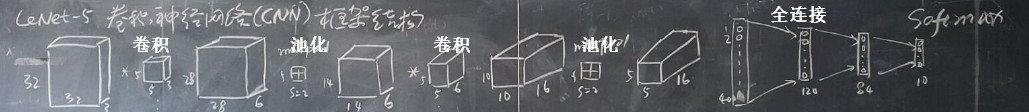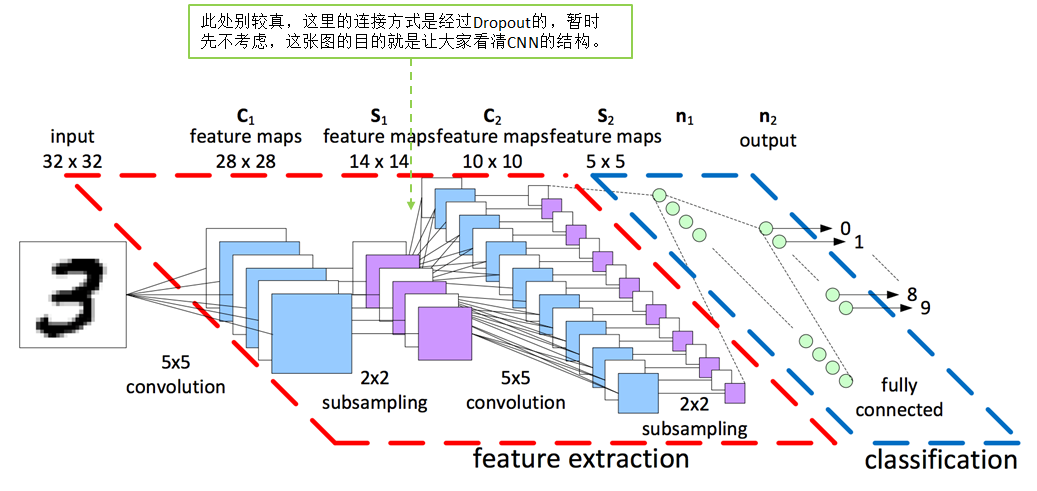## 卷积层

#### 卷积分的知识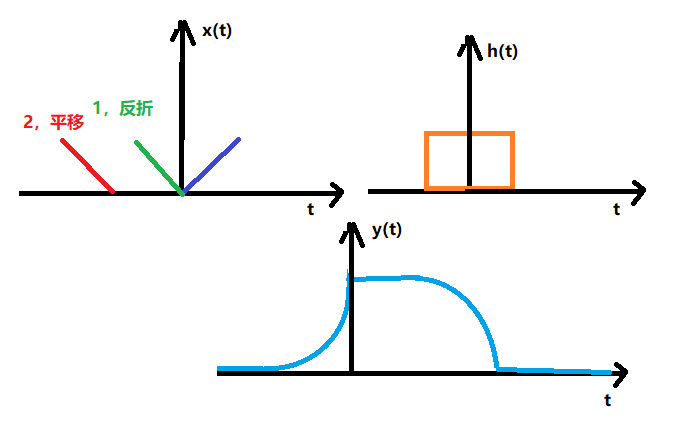#### 过程: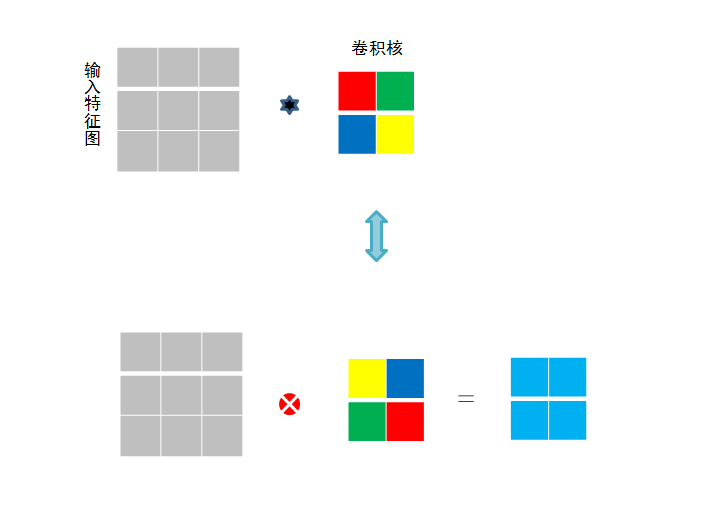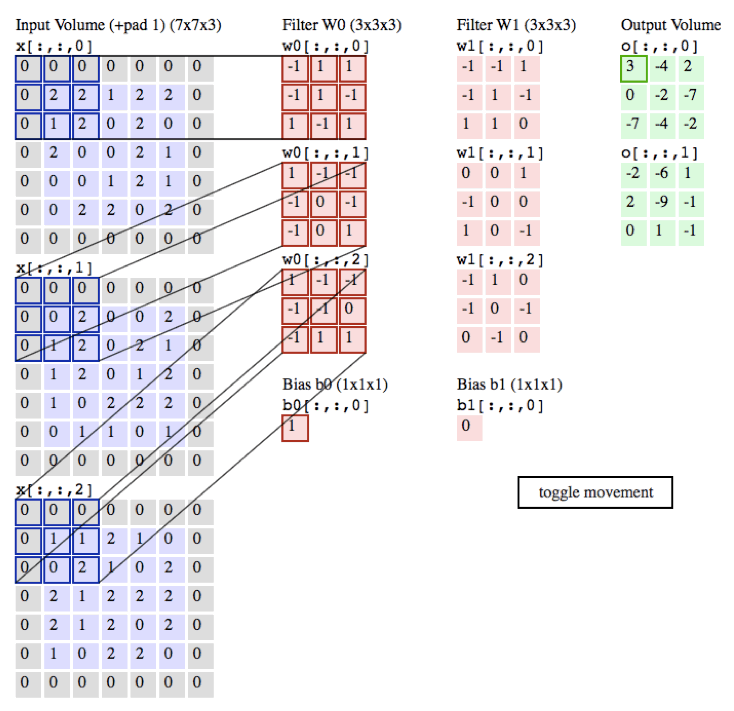#### 作用: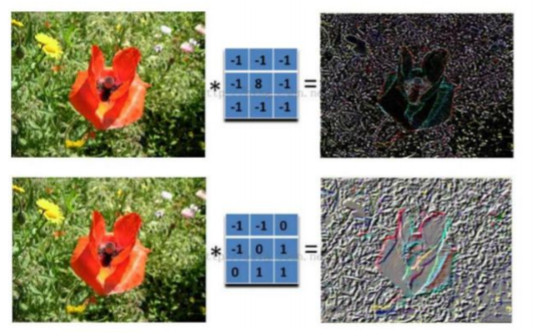#### 真实做了什么?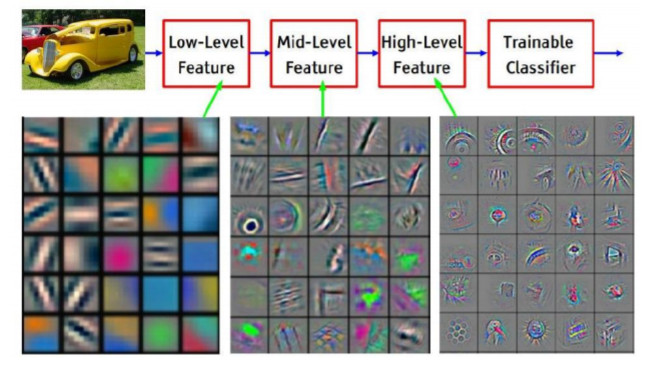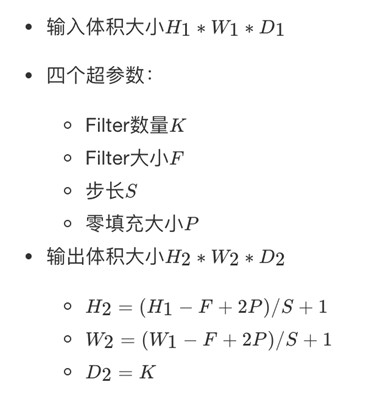# 卷积函数def conv_fun(cache):    x, w, b = cache["a"], cache["w"], cache["b"]    pad, stride = cache["pad"], cache["stride"]    N, C, H, W = x.shape    F, C, HH, WW = w.shape    # numpy提供的可以填充0的api,constant代表用一样的值填充前两维不填,后两维各自填充pad行    x_padded = np.pad(x, ((0, 0), (0, 0), (pad, pad), (pad, pad)), mode='constant')    H_new = int((H + 2 * pad - HH) / stride) + 1    W_new = int((W + 2 * pad - WW) / stride) + 1    s = stride    out = np.zeros((N, F, H_new, W_new))    for i in range(N):  # ith image        for f in range(F):  # fth filter            for j in range(H_new):                for k in range(W_new):                    out[i, f, j, k] = np.sum                    (x_padded[i, :, j * s:(HH + j * s), k * s:(WW + k * s)] * w[f]) +b[f]    return out

## 池化层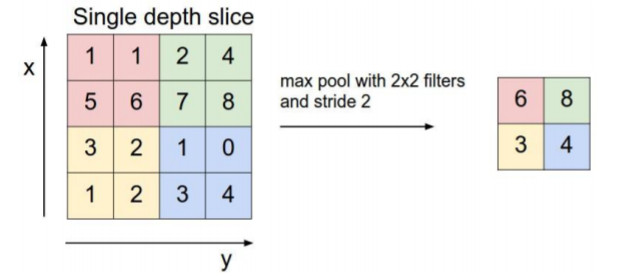# 前向池化def max_pool_forward(cache):    x, HH, WW, s = cache["net"], cache["HH"], cache["WW"], cache["s"]    N, C, H, W = x.shape    H_new = 1 + int((H - HH) / s)    W_new = 1 + int((W - WW) / s)    out = np.zeros((N, C, H_new, W_new))    for i in range(N):        for j in range(C):            for k in range(H_new):                for l in range(W_new):                    # 定位到某个窗口                    window = x[i, j, k * s:HH + k * s, l * s:WW + l * s]                    # 找到该窗口的最大值，然后赋值                    out[i, j, k, l] = np.max(window)    return out

## ReLU层

http://playground.tensorflow.org/

# Relu函数def Relu(x):    return np.maximum(0, x)

## 全连接层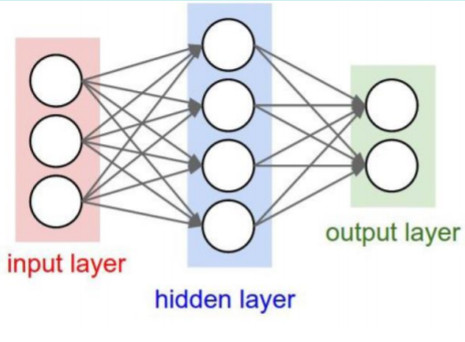# 全连接def fc(net, w, b):    N = net.shape    # 把每个像素提取出来    x_row = net.reshape(N, -1)    out = np.dot(x_row, w) + b    return out

## CNN反向传播的不同之处

1. 池化层没有激活函数。这个问题倒比较好解决，我们可以令池化层的激活函数为σ(z)=z，即激活后就是自己本身。这样池化层激活函数的导数为1。

2. 池化层在前向传播的时候，对输入进行了压缩，那么我们向前反向推导上一层的误差时，需要做upsample处理

3. 卷积层是通过张量卷积，或者说若干个矩阵卷积求和而得到当前层的输出，这和一般的网络直接进行矩阵乘法得到当前层的输出不同。这样在卷积层反向传播的时候，上一层误差的递推计算方法肯定有所不同。

4. 对于卷积层，由于W使用的运算是卷积，那么由该层误差推导出该层的所有卷积核的W,b的方式也不同。

## 池化层的反向传播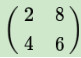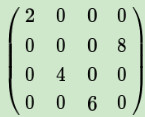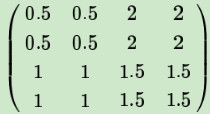## 卷积层的反向传播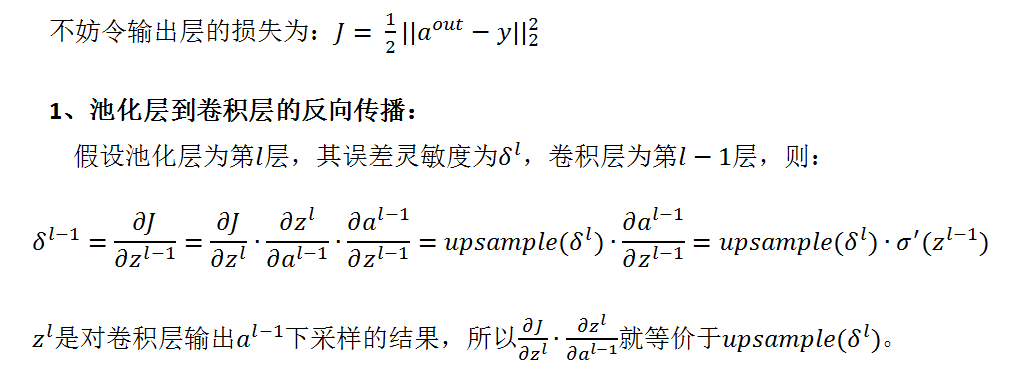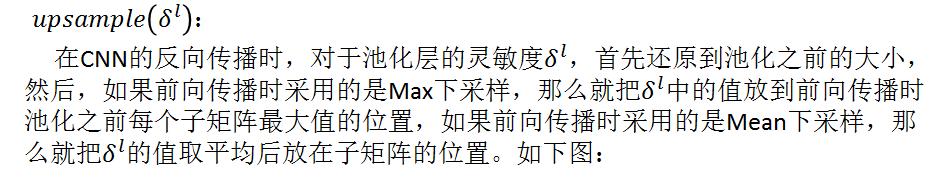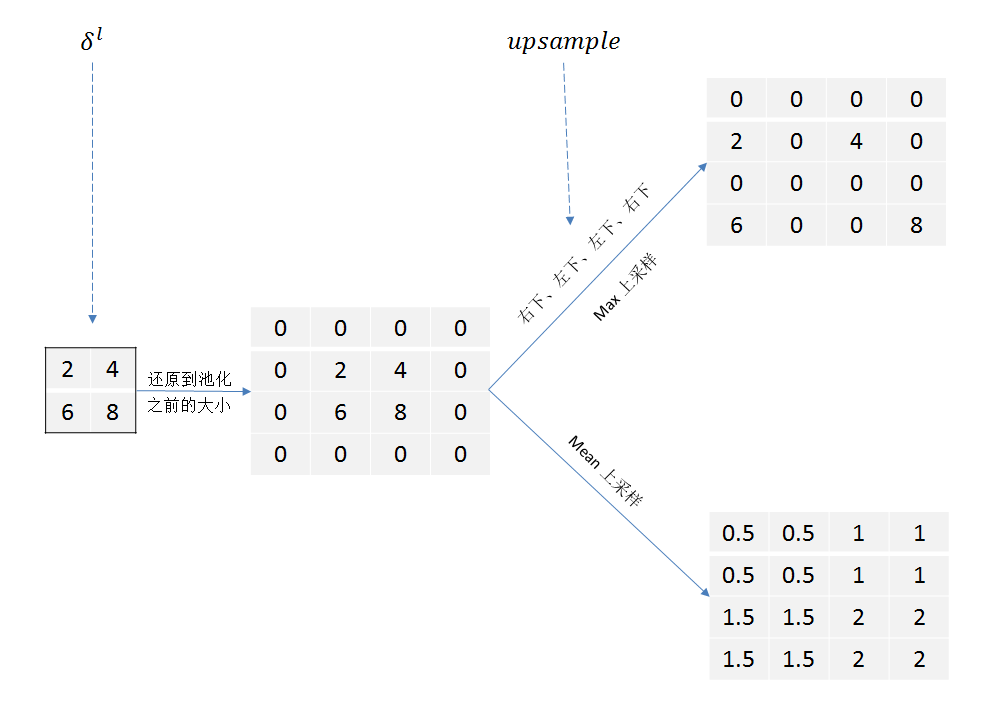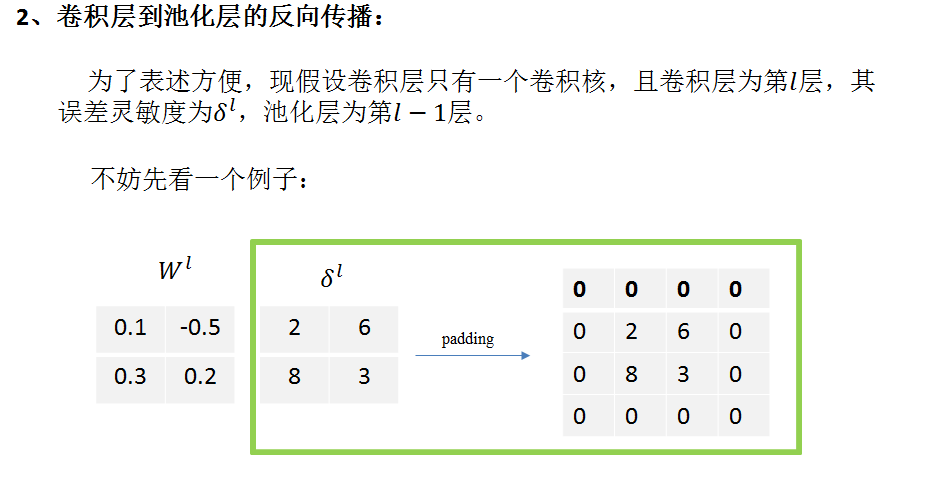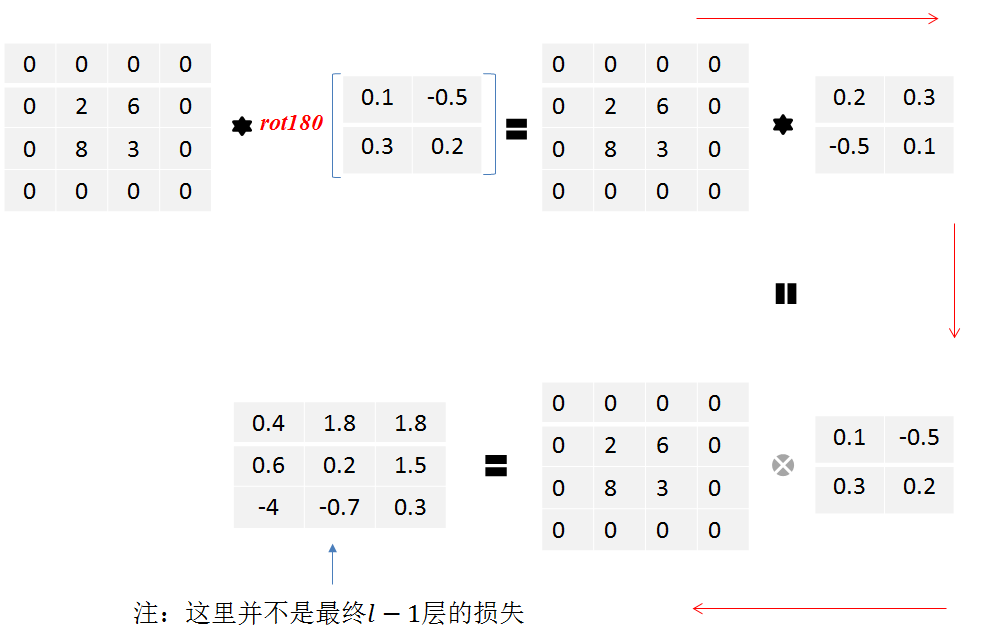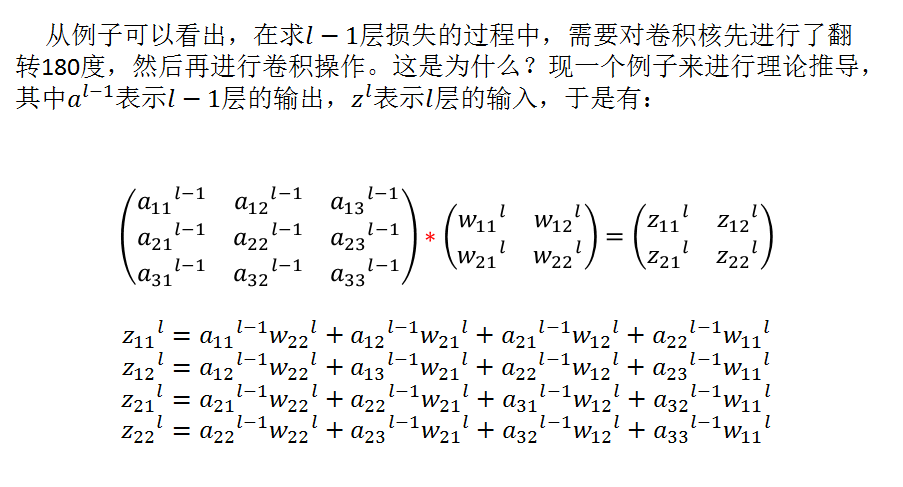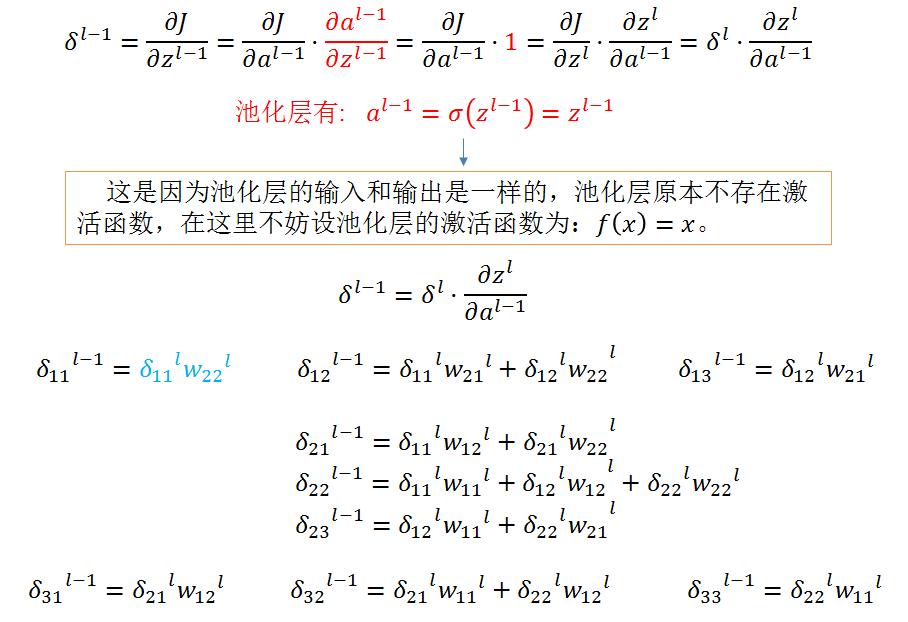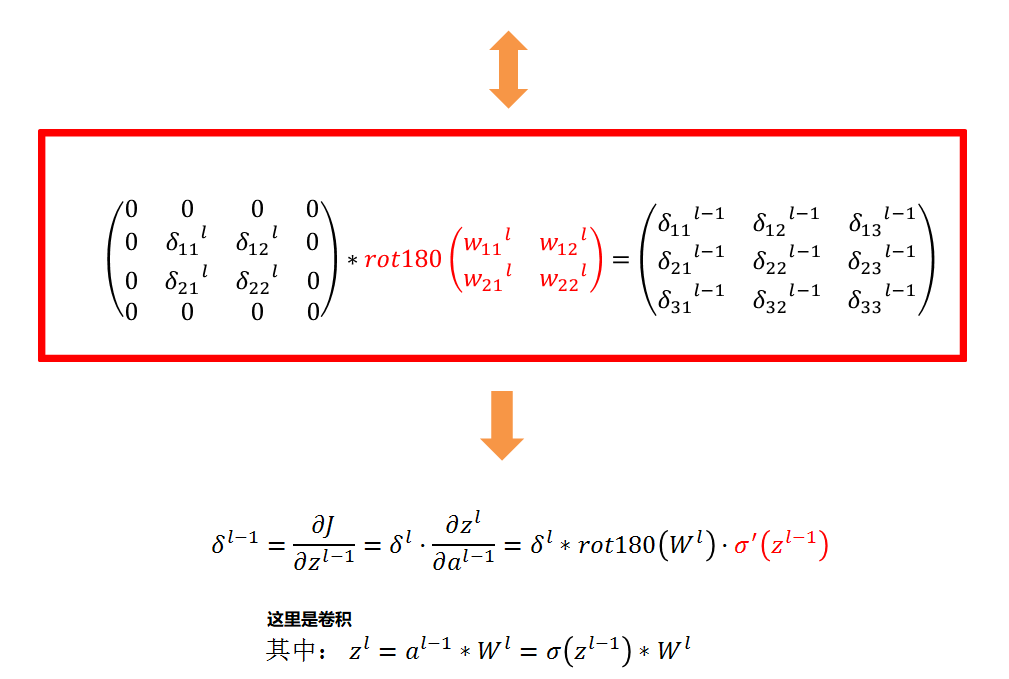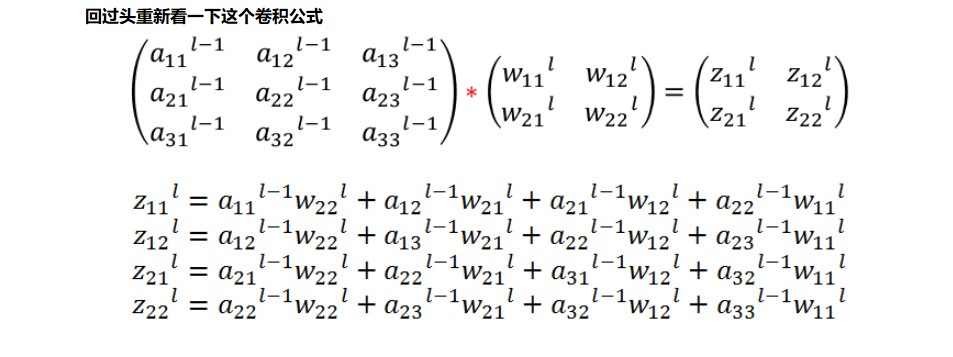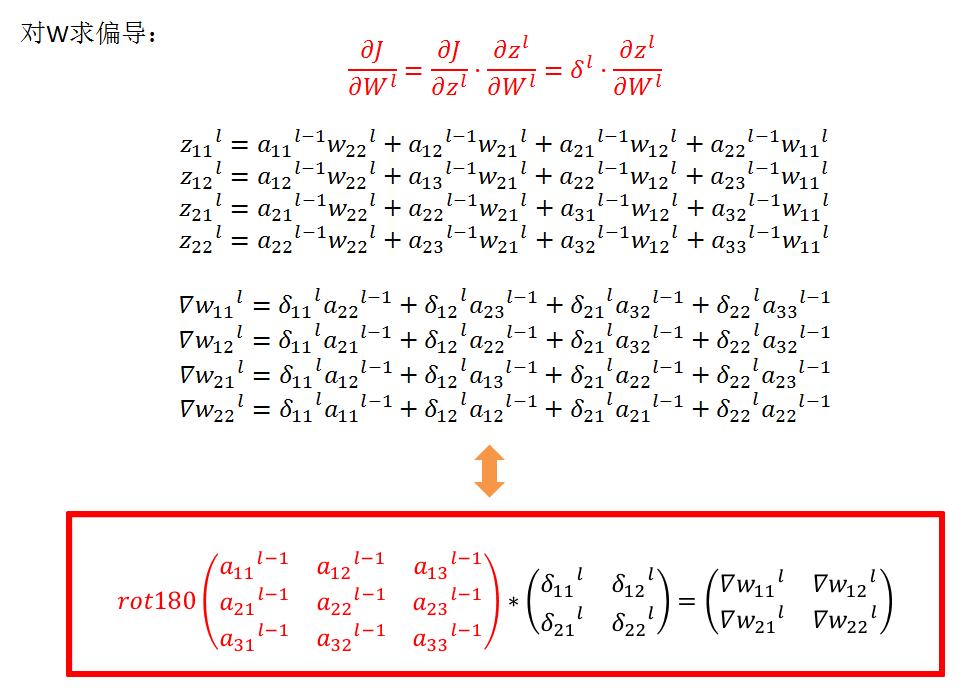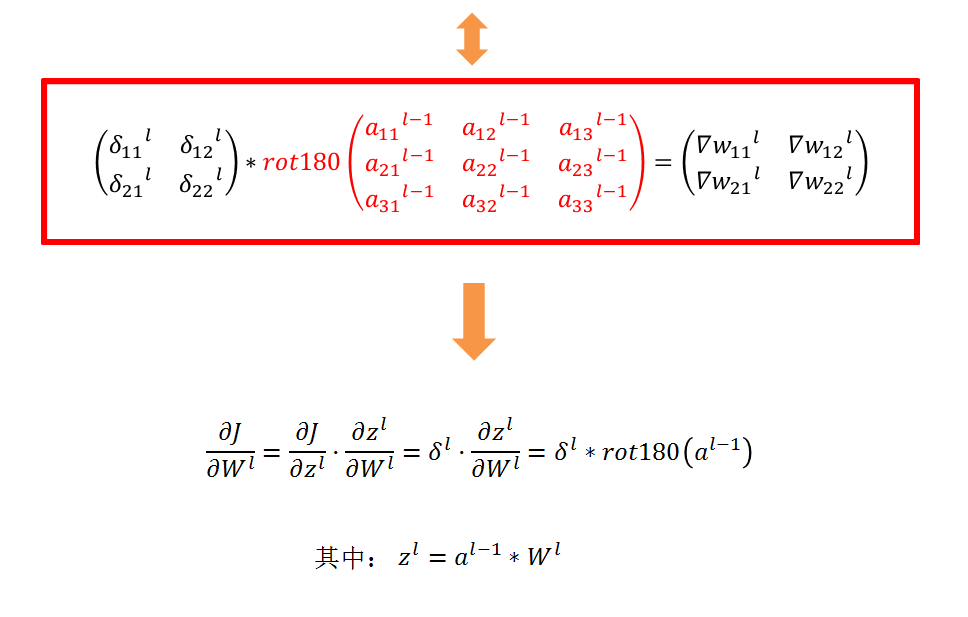## 吴恩达笔记中的推导:

### 卷积神经网络中的反向传播(可选/非梯度)

#### Convolutional layer backward pass

1. 计算dA:

da_prev_pad[vert_start:vert_end, horiz_start:horiz_end, :] +=,W[:,:,:,c] * dZ[i, h, w, c]
1. 计算dW: 这是计算dWc (dWc是一个过滤器的导数)关于损失的公式:

其中aslice是原样本的一个窗口。因此，这就得到了W关于这个窗口的梯度。这是相同的W，我们将所有这些梯度相加得到dW。

在代码中，在合适的for循环中，这个公式可以转化为:

dW[:,:,:,c] += a_slice * dZ[i, h, w, c]
2. 计算db: 这是计算db对于一个过滤器Wc的代价的公式:

正如您之前在基本神经网络中看到的，db是通过求和dZ来计算的。在本例中只需对conv输出(Z)的所有梯度求和。

在代码中，在合适的for循环中，这个公式可以转化为:

db[:,:,:,c] += dZ[i, h, w, c]

def conv_backward(dout, cache):
x, w, b = cache["a"], cache["w"], cache["b"]
F, C, HH, WW = w.shape
N, C, H, W = x.shape
H_new = 1 + int((H + 2 * pad - HH) / stride)
W_new = 1 + int((W + 2 * pad - WW) / stride)
​
dx = np.zeros_like(x)
dw = np.zeros_like(w)
db = np.zeros_like(b)
​
s = stride
​
for i in range(N):  # ith image
for f in range(F):  # fth filter
for j in range(H_new):
for k in range(W_new):
window = x_padded[i, :, j * s:HH + j * s, k * s:WW + k * s]
# db = dout //  dw=dout*x // dx = dout*w
db[f] += dout[i, f, j, k]
dw[f] += window * dout[i, f, j, k]
dx_padded[i, :, j * s:HH + j * s, k * s:WW + k * s]
+= w[f] * dout[i, f, j, k]

posted @ 2019-08-22 21:11  Timcode  阅读(...)  评论(...编辑  收藏